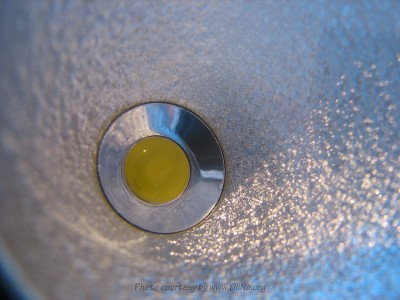# OliNo

Renewable Energy

## CDE Technology BV Led Spot MR16 10W Warm White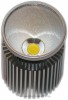presents a led spotlight with some power. With its 10 W a lot of warm white light is generated.

This article shows the measurement results. Many parameters are also found in the Eulumdat file.

See this overview for a comparison with other light bulbs.

### Summary measurement data

parameter meas. result remark
Color temperature 3332 K Warm white (on the cold site of it)
Luminous intensity Iv 367 Cd
Beam angle 47 deg
Power P 10.3 W
Power Factor 0.65 For every 1 kWh net power consumed, there has been 1.2 kVAhr for reactive power.
Luminous flux 282 Lm
Luminous efficacy 27 Lm/W
CRI_Ra 69 Color Rendering Index.
Coordinates chromaticity diagram x=0.4127 and y=0.3914
Fitting 230V It uses a current driver for the leds, converting 230 V mains into current.
PAR-value 3.3 μMol/s/m2 The number of photons seen by an average plant when it is lit by the light of this light bulb. Value valid at 1 m distance from light bulb.
S/P ratio 1.3 This factor indicates the amount of times more efficient the light of this light bulb is perceived under scotopic circumstances (ow environmental light level).
D  x H external dimensions 50 x 72 mm External dimensions of the light bulb.
D luminous area 46 mm Dimensions of the luminous area (used in Eulumdat file). This is equal to the ID of the reflector end at the front of the light bulb.
General remarks The ambient temperature during the whole set of measurements was 23.5-24.5 deg C. The light bulbs gets warmer than warm-to-the-touch (60º C).

Warm up effect: during the warm up time the illuminance decreased with 22 % and the consumed power decreased with 4 %.

Voltage dependency: the power consumption and illuminance do not vary when the voltage is varied from 200 – 250 V.

The dim-function of the current driver is tested. The three pre-sets seem to work well.

Measurement report (PDF)Eulumdat fileRight click on icon and save the file.

### Overview table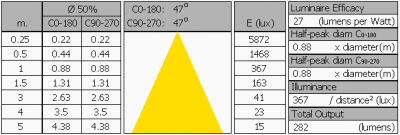The overview table is explained on the OliNo website. Please note that this overview table makes use of calculations, use this data with care as explained on the OliNo site.

### Eulumdat light diagram

This light diagram below comes from the program Qlumedit, that extracts these diagrams from an Eulumdat file. It is explained on the OliNo site.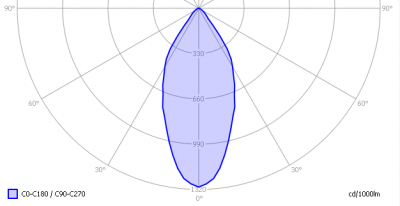The light diagram giving the radiation pattern.

It indicates the luminous intensity around the light bulb. This light bulb has the same light diagram for the C0-C180 as for the C90-C270 plane since it has symmetry over is height axis.

### Illuminance Ev at 1 m distance, or luminous intensity Iv

Herewith the plot of the averaged luminous intensity Iv as a function of the inclination angle with the light bulb.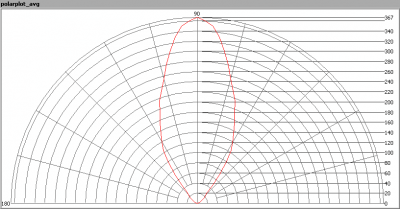The radiation pattern of the light bulb.

This radiation pattern is the average of the light output of the light diagram given earlier. Also, in this graph the luminous intensity is given in Cd.

These averaged values are used (later) to compute the lumen output.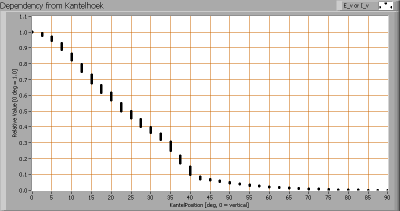Intensity data of every measured turn angle at each inclination angle.

This plot shows per inclination angle the intensity measurement results for each turn angle at that inclination angle. There normally are differences in illuminance values for different turn angles. However for further calculations the averaged values will be used.

When using the average values per inclination angle, the beam angle can be computed, being 47 degrees. This is the average value but as the light bulb has a symmetry over its height axis, this angle is valid for all turn-angles the light bulb is looked at.

### Luminous flux

With the averaged illuminance data at 1 m distance, taken from the graph showing the averaged radiation pattern, it is possible to compute the luminous flux.

The result of this computation for this light spot is a luminous flux of 282 Lm.

### Luminous efficacy

The luminous flux being 282 Lm, and the power of the light bulb being 10.3 W, yields a luminous efficacy of 27 Lm/W.

A power factor of 0.65 means that for every 1 kWh net power consumed, a reactive component of 1.2 kVAr was needed.

 Light bulb voltage (used on power supply!) 230 VAC Light bulb current 69 mA Power P 10.3 W Apparent power S 15.9 VA Power factor 0.65

Of this light bulb the voltage across ad the resulting current through it+its constant current driver power supply are measured and graphed. See the OliNo site how this is obtained.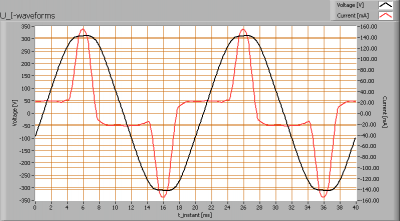Voltage across and current through the lightbulb

The current does not have a sinusiodal form. It contains some peaks instead. The current’s phase leads the voltage’s (the peaks are coincident with the tops of the voltage waveform, but the current has a constant value different than 0 when the voltage starts its sine. All together the powerfactor is relatively low with less than 0.7.

Also the power spectrum of the current is determined.Current power spectrum in % of the first harmonic (50 Hz).

The peaks in the current and the steep edges result in the appearance of the higher harmonics.

### Temperature measurements light bulb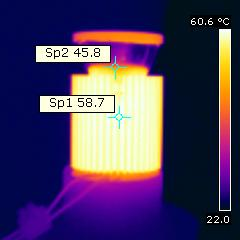Temperature measurement after warm up period

 status lamp 30 minuten warmed up ambient temperature 23 ºC camera Flir B-CAM Western S emissivity 0.95(1) measurement distance 0.4 m IFOVgeometric 1.5 mm NETD (thermal sensitivity) 100 mK

(1) The emissivity has been set such that the measured temperature i the same as when measured with a fast temperature contact probe.

The light bulb gets warmer than warm-to-the-touch (60 ºC). The outer side of the reflector also gets warm, about 45 ºC.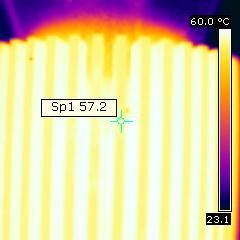Temperature of the heat sink

 emissivity 1.0(1) measurement distance 0.13 m IFOVgeometric 0.5 mm

The heat sink gets above 57 ºC. This is the temperature measured on the outside part of the heat sink. The ribs are 1 mm wide, so with an IFOVgeometric of 0.5 mm (equivalent to IFOVmeasurement of 1.5 mm) the measurement result is an average of the temperature of the outer part of the heat sink and the inner part. A camera with a higher resolution is needed to discriminate between the outer temperature and the inner.

### Color temperature and Spectral power distribution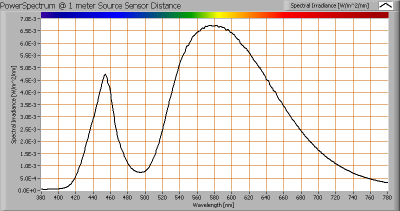The spectral power distribution of this light bulb, energies on y-axis valid at 1 m distance.

The measured color temperature is about 3325 K which is warm white.

This color temperature is measured straight underneath the light bulb. Below a graph showing the color temperature for different inclination angles.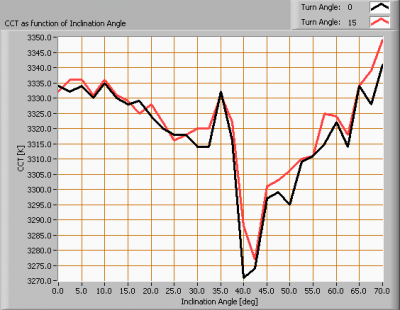Color temperature as a function of inclination angle.

The measurement of CCT has been stopped when less than 5 lux (low illumination value) was measured or when the inclination angle was 90 degrees. With this lamp at 70 degree inclination angle less than 5 lux was measured (at the specific measurement distance).

The maximum beam angle is 47 degrees, meaning at an inclination angle of 23.5 degrees there is 50 % left of the initial illuminance value at 0 degrees. Most of the light energy is within this area. In this area the color temperature stays within 1 % of its value at 0 degrees inclination angle.

### PAR value and PAR spectrum

To make a statement how well the light of this light bulb is for growing plants, the PAR-area needs to be determined. See the OliNo website how this all is determined and the explanation of the graph.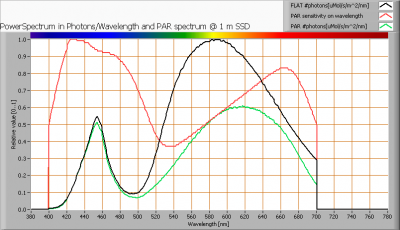The photon spectrum, then the sensitivity curve and as result the final PAR spectrum of the light of this light bulb

The PAR number for this light results in 3.3 μMol/s/m2. This value is valid at 1 m distance from the light bulb and valid roughly inside its beam angle.

The PAR efficiency is 64 % (valid for the PAR wave length range of 400 – 725 nm). So maximally 64 % of the total of photons in the light is effectively used by the average plant (since the plant might not take 100 % of the photons at the frequency where its relative sensitivity is 100 %).

### S/P ratio

The S/P ratio and measurement is explained on the OliNo website. Here the results are given.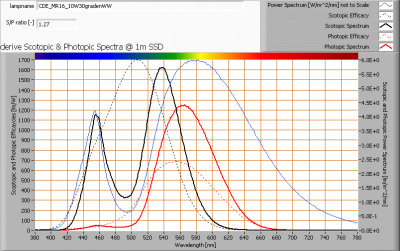The power spectrum, sensitivity curves and resulting scotopic and photopic spectra (spectra energy content defined at 1 m distance).

The S/P ratio is 1.3.

### Chromaticity diagram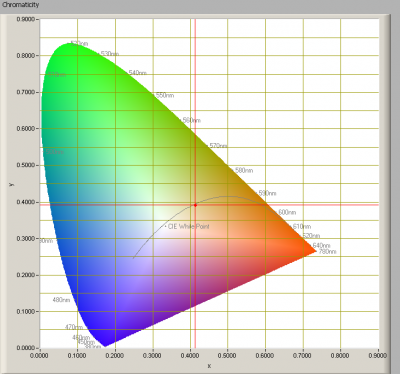The chromaticity space and the position of the lamp’s color coordinates in it.

The light coming from this lamp is close to the Planckian Locus (the black path in the graph).

Its coordinates are x=0.4127 and y=0.3914.

### Color Rendering Index (CRI) or also Ra

Herewith the image showing the CRI as well as how well different colors are represented (rendered). The higher the number, the better the resemblance with the color when a black body radiator would have been used (the sun, or an incandescent lamp).

Each color has an index Rx, and the first 8 indexes (R1 .. R8) are averaged to compute the Ra which is equivalent to the CRI.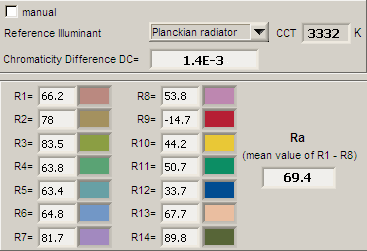CRI of the light of this lightbulb.

The value of 69 is lower than 80 which is considered a minimum value for indoor usage.

Note: the chromaticity difference is 0.0014 indicates the distance to the Planckian Locus. Its value is lower than 0.0054, which means that the calculated CRI result is meaningful.

### Voltage dependency

The dependency of a number of lamp parameters on the lamp voltage is determined. For this, the lamp voltage has been varied and its effect on the following light bulb parameters measured: illuminance E_v [lx], the lamppower P [W], the (Correlated) Color Temperature [K] and the luminous efficacy [Lm/W].Lamp voltage dependencies of certain light bulb parameters, where the value at 230 V is taken as 100 %.

The consumed power varies < 2 % and the illuminane doesn’t vary when the voltage varies between 200-250 V. This variation is insignificant.

When the voltage at 230 V varies with + and – 5 V, then the illuminance varies within 0.1 %, so when abrupt voltage changes occur this effect is not visible in the illuminance output.

### Warm up effects

After switch on of a cold lamp, the effect of heating up of the lamp is measured on illuminance E_v [lx], color temperature CT or correlated color temperature CCT [K], the lamppower P [W] and the luminous efficacy [lm/W].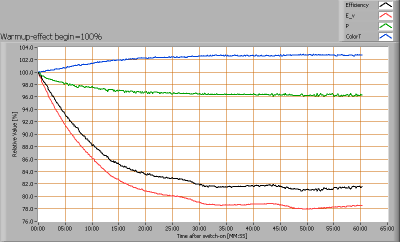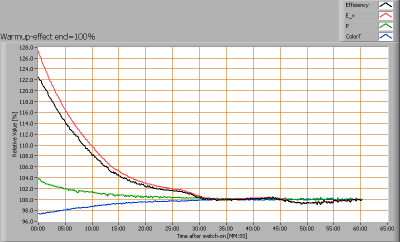Effect of warming up on different light bulb parameters. At top the 100 % level is put at begin, and at bottom at the end.

The warm-up time is about 25 minutes.

During this time the illuminance value and the consumed power decrease with 22 % and 4 % respectively.

The small variations in illuminance at the end of the measurement time come from the variations in ambient temperature (23.5 – 24.5 ºC).

### Dim-functionality

The used current source enables dimming the connected light bulbs by switching off and on within 2 seconds. The dim-presets are 100 %, 33 % and 66 %.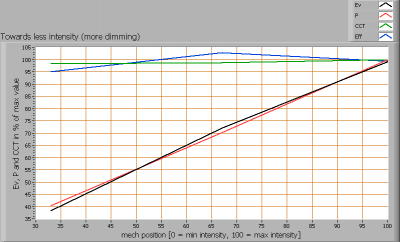Lamp parameters dependent on the dim-position

The consumed power and the illuminance values are nicely linear with the dim-presets; the illuminance follows the specified 33 %, 67 % and 100 % levels.

The consumed power decreases at the same speed as the illuminance does so the efficacy stays generally the same.

The color temperature decreases slightly when dimming takes place.